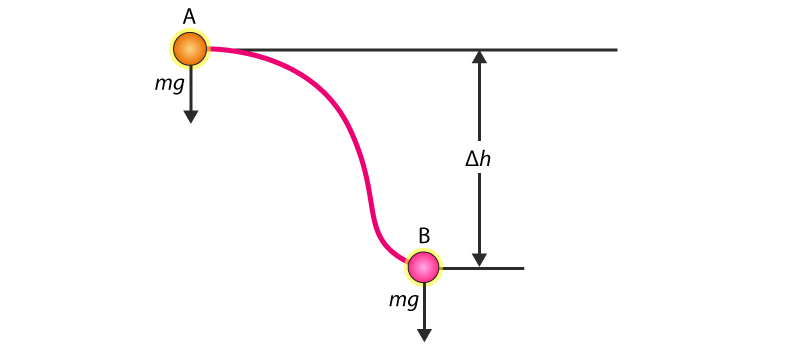# Conservative Force

A conservative force is a force done in moving a particle from one point to another, such that the force is independent of the path taken by the particle. It depends only on the initial and final position of the particle. Gravitational force and elastic spring forces are two such examples of conservation forces.

## What is Conservative Force?

As the name suggests, conservative force conserves energy. It follows the law of conservation of energy. Many forces in nature that we know of like the magnetic force, electrostatic force, gravitational force, etc. are a few examples of a conservative force. Let us understand the concept better with the help of the following example.Gravitational force acting on a particle

In the given image, the gravitational force acting on the particle has a magnitude equal to mg, where m is the mass of the substance and g is the acceleration due to gravity. The particle moves from point A to point B, and its vertical displacement is given by Δh. The blue curve in the image represents the arbitrary path traveled by the body due to the influence of other forces acting on the body. But the arbitrary path is of no consideration to the force of gravity as it is unaffected by them and therefore can be treated independently. The force of gravity is only dependent on the vertical displacement.

The total work done by gravity on the body is given as follows:

 Wg = -mg (Δh)

Where,

•  Δh is the difference between the final position (at point B) and the initial position (at point A)
• g is the acceleration due to gravity
• m is the mass of the body

No matter how complicated the path taken by the particle might be, we can easily find out the work done by gravity on the particle using the above expression just by knowing the vertical displacement. From this, we can conclude that the gravitational force doesn’t depend on the path taken but only depends on the initial and final position. Hence, the gravitational force is a conservative force.

### Properties of Conservative Forces

If a force has the following properties, then it is said to be a conservative force.

• When the force only dependent on the initial and final position irrespective of the path taken.
• In any closed path, the work done by a conservative force is zero.
• The work done by a conservative is reversible.

## What is Non-Conservative force?

A non-conservative force is a force for which the work done depends on the path taken. Friction is an example of a non-conservative force. A force is said to be a non-conservative force if it results in the change of mechanical energy, which is nothing but the sum of potential and kinetic energy. The work done by a non-conservative force adds or removes mechanical energy. For example, when work is done by friction, thermal energy is dissipated. The energy lost cannot be fully recovered.

### Properties of Non-Conservative Forces

It has the opposite properties of conservative forces. The properties are given below:

• It is path dependent therefore it also depends on the initial and final velocity.
• In any closed path, the total work done by a non-conservative force is not zero.
• The work done by a non-conservative is irreversible.Stay tuned to BYJU’S to learn more interesting concepts like conservation of mechanical energy. If you wish to learn more physics concepts with the help of interactive video lessons, download BYJU’S – The Learning App.

Test Your Knowledge On Conservative Force!